Courses

# NCERT Solutions(Part - 2) - Comparing Quantities Class 7 Notes | EduRev

## Mathematics (Maths) Class 7

Created by: Praveen Kumar

## Class 7 : NCERT Solutions(Part - 2) - Comparing Quantities Class 7 Notes | EduRev

The document NCERT Solutions(Part - 2) - Comparing Quantities Class 7 Notes | EduRev is a part of the Class 7 Course Mathematics (Maths) Class 7.
All you need of Class 7 at this link: Class 7

Exercise 8.3

Question 1: Tell what is the profit or loss in the following transactions. Also find profit percent or loss percent in each case.

(a) Gardening shears bought for Rs 250 and sold for Rs 325.

(b) A refrigerator bought Rs12,000 and sold at Rs 13,500.

(c) A cupboard bought for Rs 2,500 and sold at Rs 3,000.

(d) A skirt bought for Rs 250 and sold at Rs 150.

Answer 1: (a) Cost price of gardening shears = Rs 250

Selling price of gardening shears = Rs 325
Since, S.P. > C.P., therefore here is profit.
∴ Profit = S.P. - C.P. = Rs 325 - Rs 250 = Rs 75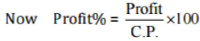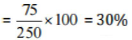(b) Cost price of refrigerator = Rs 12,000
Selling price of refrigerator = Rs 13,500
Since, S.P. > C.P., therefore here is profit.
∴ Profit = S.P. - C.P. = Rs 13500 - Rs 12000 = Rs 1,500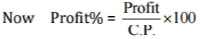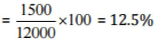Therefore, Profit = Rs 1,500 and Profit% = 12.5%

(c) Cost price of cupboard = Rs 2,500
Selling price of cupboard = Rs 3,000
Since, S.P. > C.P., therefore here is profit.
∴ Profit = S.P. - CP. = Rs 3,000 - Rs 2,500 = Rs 500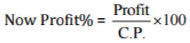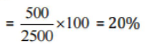Therefore, Profit = Rs 500 and Profit% = 20%

(d) Cost price of skirt = Rs 250
Selling price of skirt = Rs 150
Since, C.P. > S.P., therefore here is loss.
∴ Loss = CP, - S.P. = Rs 250 - Rs 150 = Rs100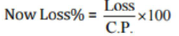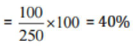Therefore, Profit = Rs 100 and Profit% = 40%

Question 2: Convert each part of the ratio to percentage:

(a) 3 : 1

(b) 2 : 3 : 5

(c) 1 : 4

(d) 1 : 2 : 5

Total part =3 + 1= 4
Therefore, Fractional part = 3/4 : 1/4
⇒ Percentage of parts =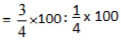⇒ Percentage of parts = 75% : 25%

(b) 2 : 3 : 5

Total part = 2 + 3 + 5 = 10

Therefore, Fractional part =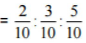⇒ Percentage of parts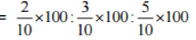⇒ Percentage of parts = 20% : 30% : 50%

(c) 1:4

Total part =1 + 4 = 5

Therefore, Fractional part = 1/5 : 4/5
⇒ Percentage of parts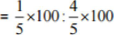⇒ Percentage of parts = 20%: 00%

(d) 1 : 2 : 5
Total part = 1+ 2 + 5 = 8

Therefore, Fractional part =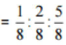⇒ Percentage o f parts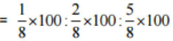⇒ Percentage of parts = 12.5% : 25% : 62.5%

Question 3: The population of a city decreased from 25,000 to 24,500. Find the percentage decrease.

Answer 3: The decreased population of a city from 25,000 to 24,500.

Population decreased = 25,000 - 24,500 = 500

Decreased Percentage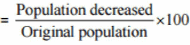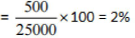Hence, the percentage decreased is 2%.

Question 4: Arun bought a car for Rs 3,50,000. The next year, the price went up to Rs 3,70,000. What was the percentage of price increase?

Answer 4: Increased in price of a car from Rs 3,50,000 to Rs 3,70,000.

Amount change = Rs 3,70,000 - Rs 3,50,000 = Rs 20,000.

Therefore, Increased percentage =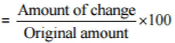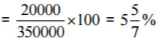Hence, the percentage of price increased is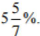Question 5: I buy a T.V. for Rs 10,000 and sell it at a profit of 20%. How much money do I get for it?

Answer 5: The cost price of T.V. = Rs 10,000

profit percent = 20% Now,
Profit = Profit% of C.P,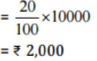Selling price = C.P. + Profit = Rs 10,000 + Rs 2,000 = Rs 12,000
Hence, he gets Rs 12,000 on selling his T.V.

Question 6: Juhi sells a washing machine for Rs 13,500. She loses 20% in the bargain. What was the price at which she bought it?

Answer 6: Selling price of washing machine = Rs. 13,500

Loss percent = 20%
Let the cost price of washing machine be Rs x.
Since, Loss = Loss% of C.P.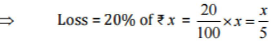Therefore, S.P. = C.P, - Loss

⇒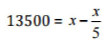⇒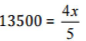⇒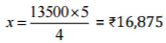Question 7: (i) Chalk contains Calcium, Carbon and Oxygen in the ratio 10:3:12. Find the percentage of Carbon in chalk.

(ii) If in a stick of chalk, Carbon is 3 g, what is the weight of the chalk stick?

Answer 7: (i) Given ratio = 10 : 3 : 12

Total part = 10 + 3 + 12 = 25

Part of Carbon = 3/25

Percentage of Carbon part in chalk =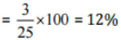(ii) Quantity of Carbon in chalk stick = 3 g
Let the weight of chalk be x g.
Then, 12 % of x = 3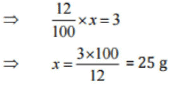Hence, the weight of chalk stick is 25 g.

Question 8: Amina buys a book for Rs 275 and sells it at a loss of 15%. How much does she sell it for?

Answer 8: The cost of a book = Rs 275

Loss percent = 15%
Loss = Loss% of C.P. = 1 5 % of Rs 275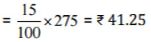Therefore, S.P. = C.P. - Loss = Rs 275 - Rs 41.25 = Rs 233.75

Hence, Amina sells a book for Rs 233.75,

Question 9: Find the amount to be paid at the end of 3 years in each case:

(a) Principal = Rs 1,200 at 12% p.a.

(b) Principal = Rs 7,500 at 5% p.a.

Answer 9: (a) Here, Principal (P) = Rs 1,200, Rate (R) = 12% p.a., Time (T) = 3 years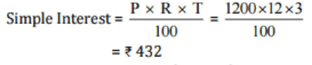Now, Amount = Principal + Simple Interest
= Rs 1200 + Rs 432
= Rs 1,632

(b) Here, Principal (P) = Rs 7,500, Rate (R) = 5% p.a., Time (T) = 3 years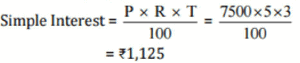Now, Amount = Principal + Simple Interest
= Rs 7,500 + Rs 1,125
= Rs 8,625

Question 10: What rate gives Rs 280 as interest on a sum of Rs 56,000 in 2 years?

Answer 10: Here, Principal (P) = Rs 56,000, Simple Interest (S.I.) = Rs 280, Time (T) = 2 years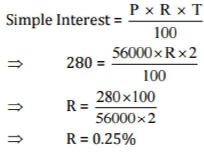Hence, the rate of interest on sum is 0.25%.

Question 11: If Meena gives an interest of Rs 45 for one year at 9% rate p.a. What is the sum she has borrowed?

Answer 11:  Simple Interest = Rs 45, Rate (R) = 9% p.a., Time (T) = 1 years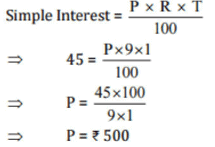Hence, she borrowed Rs 500.

Offer running on EduRev: Apply code STAYHOME200 to get INR 200 off on our premium plan EduRev Infinity!

,

,

,

,

,

,

,

,

,

,

,

,

,

,

,

,

,

,

,

,

,

;